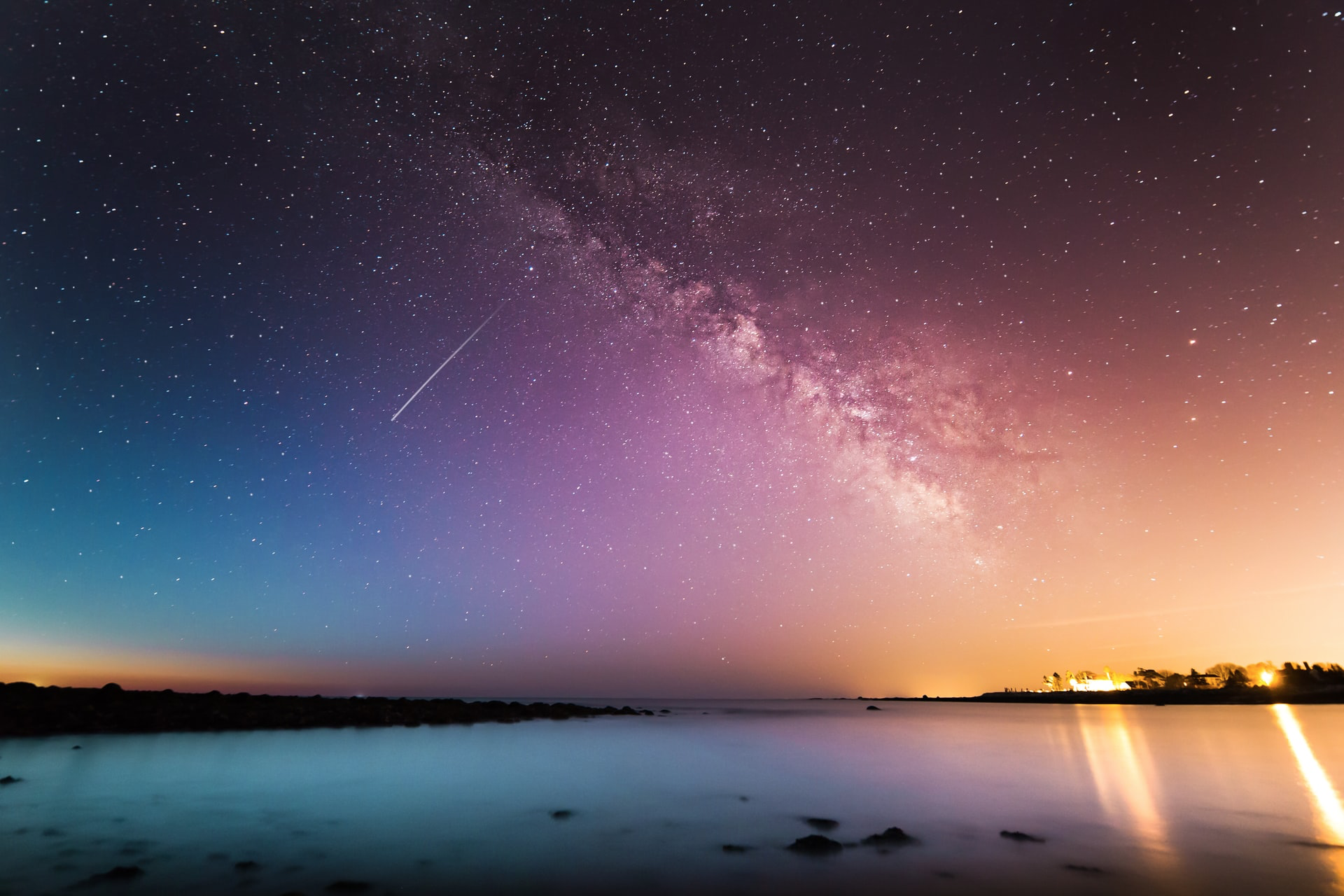Photo by Kristopher Roller on Unsplash

# A Probability Question

In any 15-minute interval, there is a 20% probability that you will see at least one shooting star. What is the probability that you see at least one shooting star in the period of an hour?

We will solve this question assuming independence of the events.

# Solution I: Intuition

## Explanation of Solution I:

For only one case:

For 4 independent cases:

# Solution II: Poisson Distribution

You can read this great TDS article by Will Koehrsen for a detailed explanation of Poisson’s distribution.

The number of shooting stars in a 15-minute time interval follows a Poisson distribution with a rate 15𝛌.

You can solve these equations using Python or this excellent Desmos calculator.

For this particular equation, we can do it manually.

It can look like that Poisson distribution is making things more complicated. Although either solution works for this simple problem, when the problem gets more complex, Poisson distribution solution works better than the intuitional one.

# Now it is your turn

Now that you know how to solve the above question, you can practice by solving a similar interview question by both intuition and Poisson distribution:

The probability of seeing a shooting star in 1 hour is 90 %. What is the probability of seeing a shooting star in 15 minutes?

# Contact me

If you want to be updated with my latest articles follow me on Medium. You can connect with me on LinkedIn, and email me at seymatas@gmail.com!

Any suggestions and comments will be very appreciated!

## More from Seyma Tas

Data Scientist, Data Educator, Blogger https://www.linkedin.com/in/seyma-tas/